# Object detection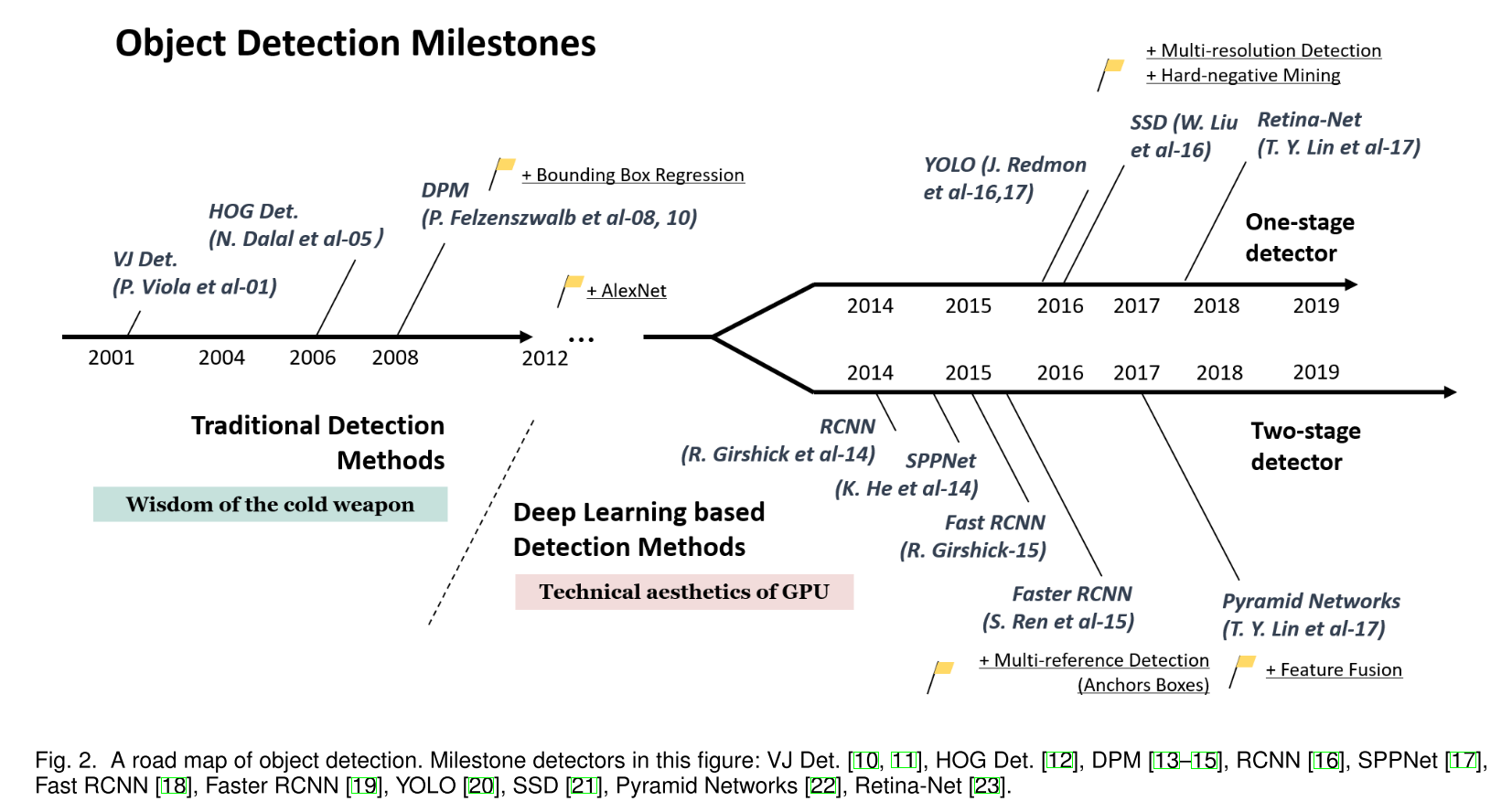◎ Object Detection in 20 Years: A Survey

2. 两阶段的候选框提取+分类的算法，首先提取出候选区域ROI，然后对它们进行深度学习方法为主的分类，如R-CNN, SPP-Net, Fast R-CNN, Faster R-CNN, R-FCN等。

算法主要组成备注
R-CNNSelective search + CNN + SVMPaper | Code
SPP-NetROI PoolingPaper | Code
Fast R-CNNSelective search + CNN + ROIPaper | Code
Faster R-CNNRPN + CNN + ROIPaper | Code
R-FCNPaper | Code
3. 一阶段的基于深度学习的回归方法，将候选框位置以及候选框的类别当作回归问题来解决，如YOLO/SSD/DenseBox等。

算法主要组成备注
YOLOAnchor boxes, YOLO-loss functionPaper(v3) | Code
SSDPaper | Code
DenseBoxPaper | Code

## Basic knowledge in Deep Learning

### Metrics

#### Confusion matrix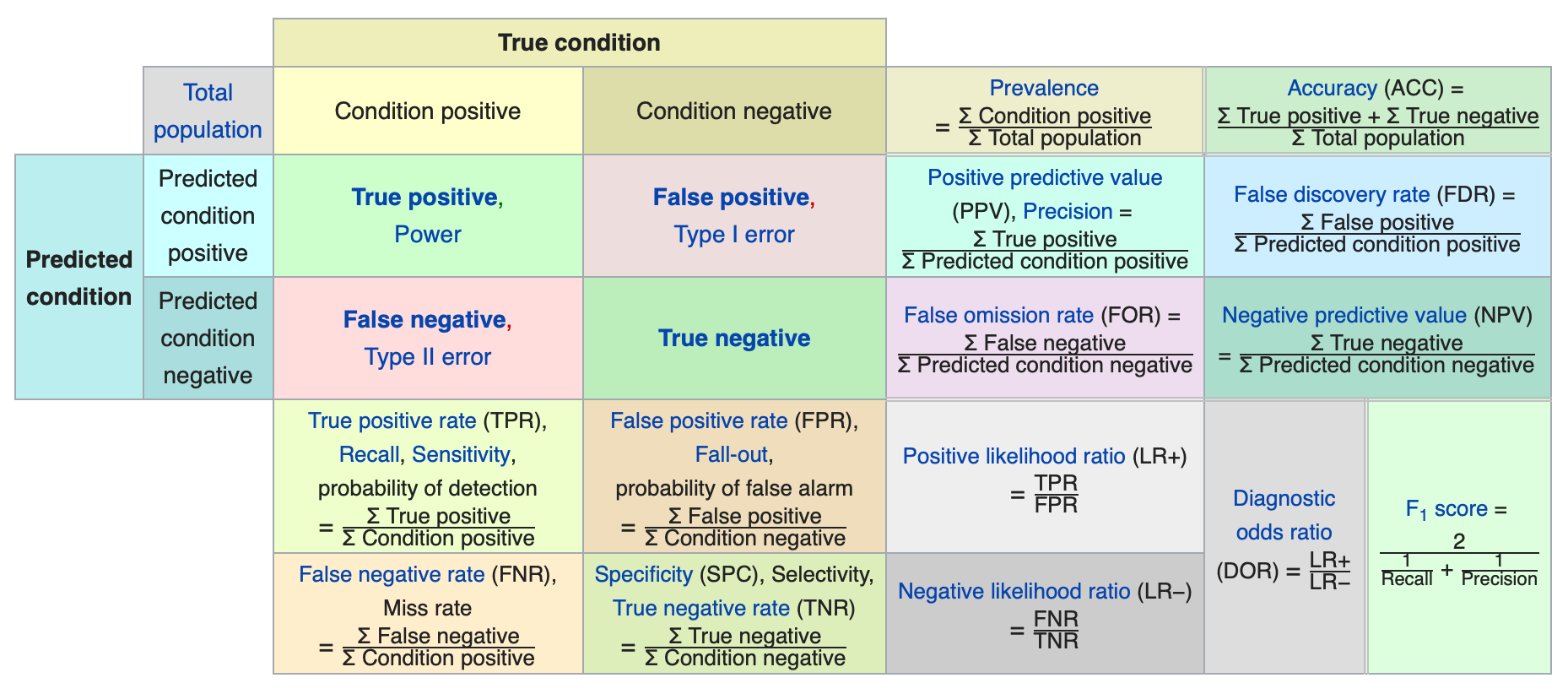• PR Curve: Precision-vs-Recall graph. The higher it is, the better the model is. The AUC is Average Precision.
• ROC Curve: TPR-vs-FPR graph at different classification thresholds. AUC stands for "Area under the Curve". Model whose predictions are 100% correct has an AUC of 1.0. ROC curve disregards sample imbalance.

N.B.

• We are often concerned with Accuracy, Precision and Recall.
• Sensitivity is also called the Recall.

Coding:

#### IoU

The rate of intersection over union between the predicted bounding box and the ground truth bounding box. WHY? To measure how accurate is the object identified in the image and to decide whether to consider the object as a true positive or a false positive. A general threshold for IoU can be 0.5.

$$IoU=\frac{\text{Area of Overlap}}{\text{Area of Union}}$$

Coding:

#### mAP

Average Precision (AP) computes the average precision for recall rate over 0 to 1. The general definition for the AP is the AUC of PR curve. $AP=\int^1_0 p(r)dr$.

Maximum precision. To smooth the PR curve, the precision value at each recall level is replaced with the maximum precision value to the right of this recall level. $p_{interp}(r)=\underset{\hat{r}>r}{max},p(\hat{r})$.

• PASCAL VOC2008 calculated an average for the 11-point interpolated AP. The recall values are sampled at 0, 0.1, 0.2, ..., 0.9 and 1.0 then the average of maximum precision values for the 11 recall values are computed. $AP=\frac{1}{11}\sum_{r\in {0, 0.1, ..., 1.0}}p_{interp}(r)$.
• For PASCAL VOC2010-2012, AP=AUC after removing zigzags:

$$AP=\sum_{r\in {r_1, r_2,..., r_N}}(r_{n+1}-r_{n})p_{interp}(r_{n+1})\\ p_{interp}(r_{n+1})=\underset{\hat{r}\geq r_{n+1}}{max},p(\hat{r})$$

• COCO mAP used a 101-point interpolated AP. AP is averaged over 10 IoU thresholds of .50: .05: .95 and over all 80 categories.

### Non Maximum Suppression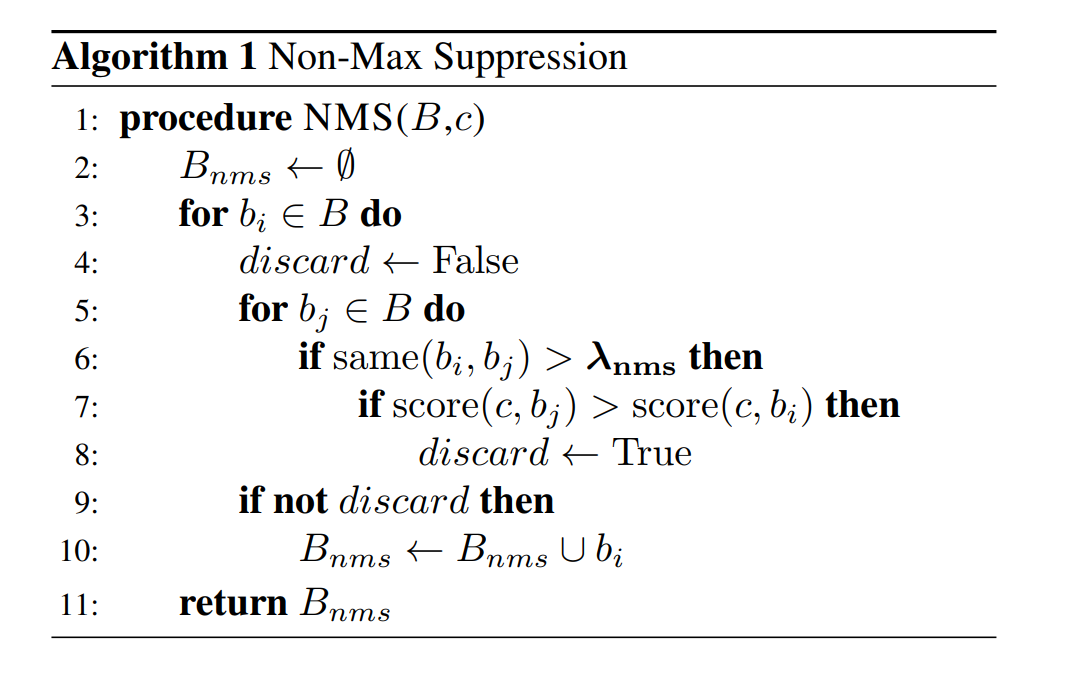◎ Hard NMS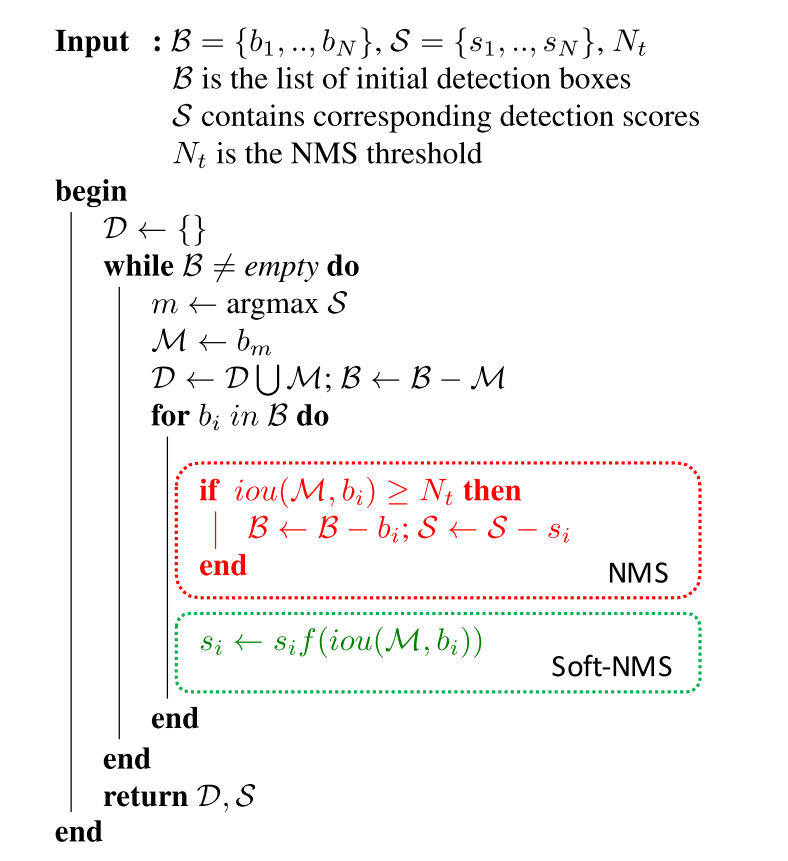◎ Hard and Soft NMS

### Dataset and splits

#### Datasets

• Training dataset: Consisted of the samples of data used to fit the model. The model learns from the training set to tune weights and biases.
• Validation dataset: Consisted of the samples of data that provide an unbiased evaluation of the model that is fit on the training dataset in the process of learning. While tuning the parameters of the model, we use the validation dataset for frequent and regular evaluation and based on the results of frequent evaluations to modify the hyperparameters. Therefore, the effects of validation set on model parameters are indirect.
• Test dataset: Consisted of the samples of data that provide an unbiased evaluation of the already learned model. The test set is used to evaluate the level of competence of the learned model.

### Hand detection using multiple proposals

In general, this paper made two contributions in hand detection domain.

• The proposing of a two-stage hand detector.
• A large dataset of images with ground truth annotations for hands.

## DL-based Single-Shot Object Detection

### YOLO

Summary:

• Unified prediction of bounding boxes.
• Network architecture.
• Design of the loss function.

### YOLOv2

Summary:

The main contributions that this paper made in the improved YOLOv2 are:

• Improved the resolution of training images.
• Applied anchor boxes (from Faster R-CNN) to predict bounding boxes.
• Replaced the fully connected layer in the output layer in YOLO with a convolutional layer.

Another contribution is that they used a new dataset combination method and joint training algorithm to train a model on more than 9000 classes.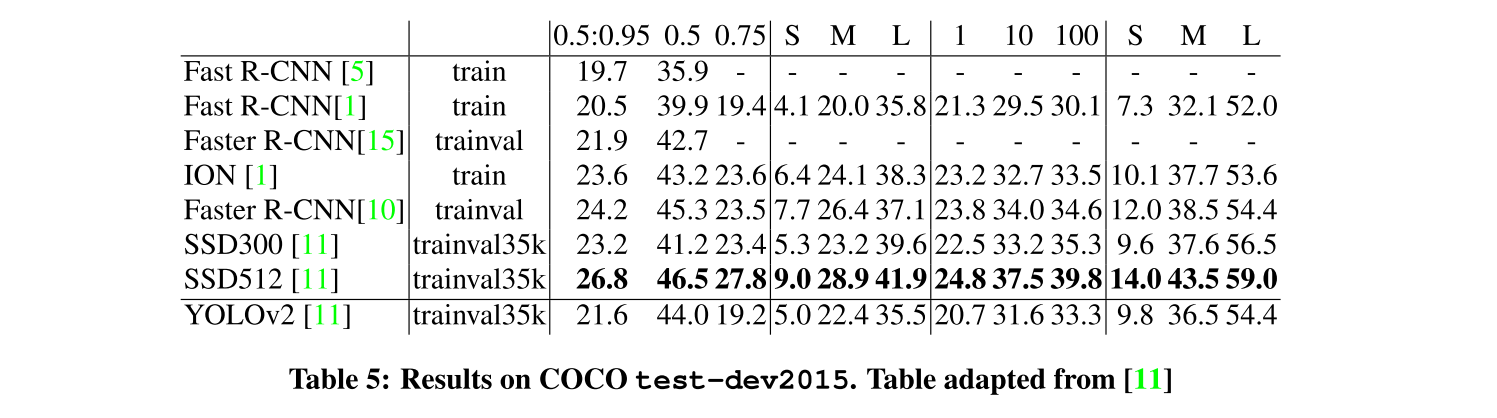### YOLOv3

Abstract:

We present some updates to YOLO! We made a bunch of little design changes to make it better. We also trained this new network that's pretty swell. It's a little bigger than last time but more accurate. It's still fast though, don't worry. At 320 × 320 YOLOv3 runs in 22 ms at 28.2 mAP, as accurate as SSD but three times faster. When we look at the old .5 IOU mAP detection metric YOLOv3 is quite good. It achieves 57.9 AP 50 in 51 ms on a Titan X, compared to 57.5 AP 50 in 198 ms by RetinaNet, similar performance but 3.8× faster. As always, all the code is online at https://pjreddie.com/yolo/.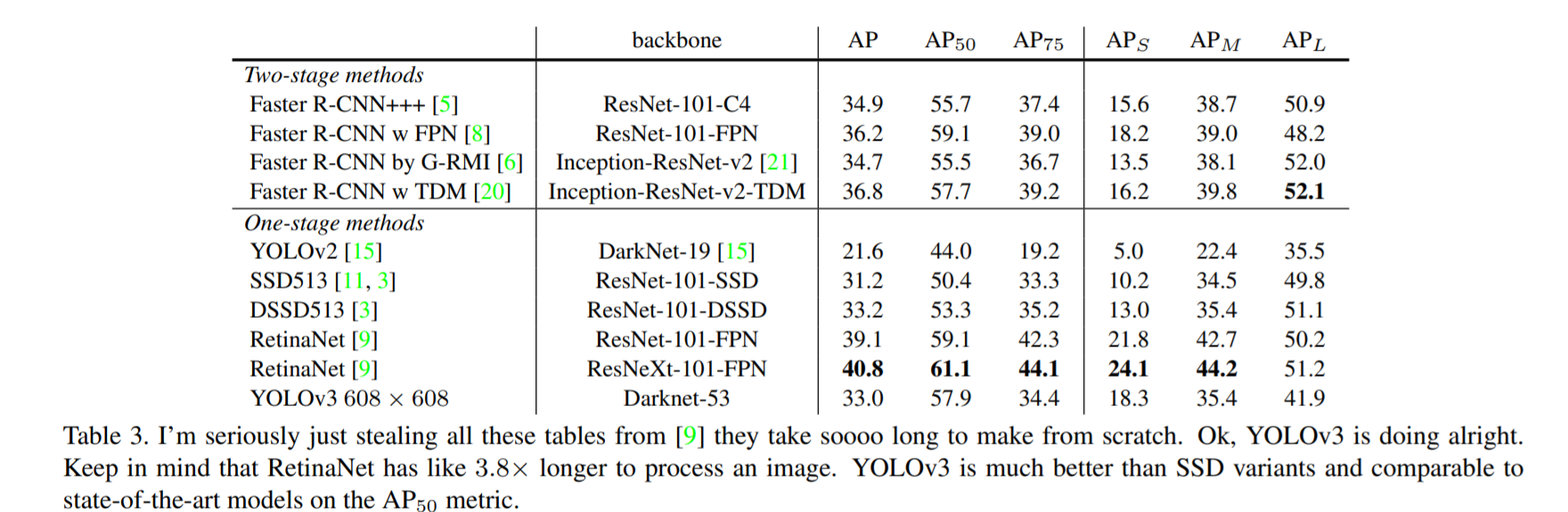### YOLOv4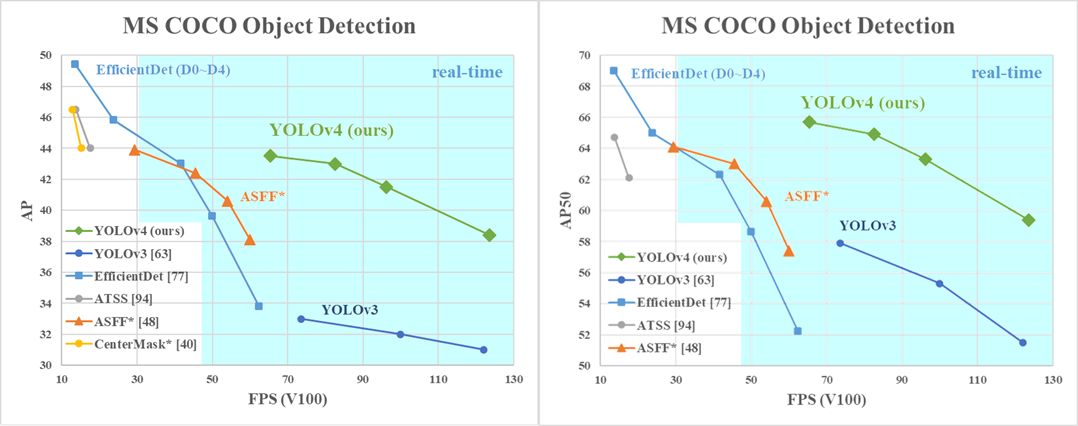## Face detection

### Viola-Jones methods

• 有比较好的表现，real-time performance
• 在实际场景(larger visual variations of human faces)中degrade很快，即使使用了更加高级的features和分类器。

## Tools & Resources

### Netron

https://github.com/lutzroeder/netron，画神经网络结构图，可以采用不同文件类型的model。以PyTorch为例，使用Netron打开我们保存的三级网络的保存文件.pkl就画出来了。

### Collection of CV Colab Notebooks

Top Computer Vision Google Colab Notebooks

Here is a list of the top google colab notebooks that use computer vision to solve a complex problem such as object detection, classification etc...

defer offscreen images based on pagespeed insights